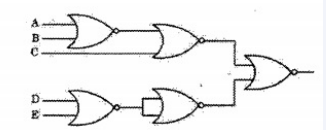ISRO | ISRO CS 2008 | Question 2

• Difficulty Level : Easy
• Last Updated : 06 Jun, 2018

The circuit shown in the following figure realizes the function(A) ( ( A + B )’ + C ) ( D’ E’ )
(B) ( ( A + B)’ + C)(D E’ )
(C) ( A + ( B + C )’) ( D’E )
(D) (A + B + C’ )( D’ E’)

Explanation: The expression can be simplified by using the DeMorgan’s theorem i.e. (A + B)’ = A’. B’ or ( A.B)’ = (A’ + B’)

(((A + B)' + C)' + ((D + E)')')'
((A + B)' + C) ((D + E)'
((A + B)' + C) (D' E')

Option (B) is correct.

Quiz of this Question
Please comment below if you find anything wrong in the above post

My Personal Notes arrow_drop_up